# Search Results (17)

View
Selected filters:
• Linear Functions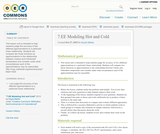Conditions of Use:
Remix and Share
Rating

This lesson unit is intended to help students judge the accuracy of two different approximations to a particular linear relationship. Students will compare two linear functions as approximations to the relationship between Celsius and Fahrenheit temperature and consider under what circumstances each of the approximations may be reasonable.

http://map.mathshell.org/download.php?fileid=1629

Subject:
Mathematics
Material Type:
Lesson
Author:
Kayla Martin
Date Added:
01/19/2017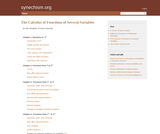Conditions of Use:
Remix and Share
Rating

This books covers the following topics: Geometry of Rn; functions from R to Rn; functions from Rn to R; functions from Rm to Rn.

Subject:
Calculus
Material Type:
Homework/Assignment
Textbook
Provider:
Furman University
Author:
Dan Sloughter
Date Added:
02/16/2011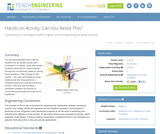Conditions of Use:
Read the Fine Print
Rating

This lab demonstrates Ohm's law as students set up simple circuits each composed of a battery, lamp and resistor. Students calculate the current flowing through the circuits they create by solving linear equations. After solving for the current, I, for each set resistance value, students plot the three points on a Cartesian plane and note the line that is formed. They also see the direct correlation between the amount of current flowing through the lamp and its brightness.

Subject:
Engineering
Physics
Material Type:
Activity/Lab
Provider:
TeachEngineering
Provider Set:
TeachEngineering
Author:
Aubrey McKelvey
Date Added:
09/18/2014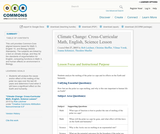Conditions of Use:
Remix and Share
Rating

This unit provides Common-Core aligned lessons based for Math 3, English 10, and Biology (NGSS Standards). The subjects are linked by a text on climate change, and they hit the standards of argumentation for English, comparing functions in Math 3, and human effects on environment in Biology.

Subject:
Applied Science
English Language Arts
Life Science
Mathematics
Material Type:
Activity/Lab
Assessment
Data Set
Diagram/Illustration
Homework/Assignment
Interactive
Lesson Plan
Primary Source
Reading
Teaching/Learning Strategy
Author:
Joanna Schimizzi
Christine Sheffler
Rob Leichner
Theodore Mueller
Yilmaz Yoruk
Date Added:
01/28/2016Conditions of Use:
Remix and Share
Rating

College Algebra is an introductory text for a college algebra survey course. The material is presented at a level intended to prepare students for Calculus while also giving them relevant mathematical skills that can be used in other classes. The authors describe their approach as "Functions First," believing introducing functions first will help students understand new concepts more completely. Each section includes homework exercises, and the answers to most computational questions are included in the text (discussion questions are open-ended).

Subject:
Algebra
Material Type:
Textbook
Provider:
Stitz-Zeager Open Source Mathematics
Author:
Carl Stitz
Jeff Zeager
Date Added:
07/15/2011Rating

This lesson unit is intended to help teachers assess how well students are able to interpret exponential and linear functions and in particular to identify and help students who have the following difficulties: translating between descriptive, algebraic and tabular data, and graphical representation of the functions; recognizing how, and why, a quantity changes per unit intervale; and to achieve these goals students work on simple and compound interest problems.

Subject:
Algebra
Measurement and Data
Material Type:
Assessment
Lesson Plan
Provider:
Shell Center for Mathematical Education
U.C. Berkeley
Provider Set:
Mathematics Assessment Project (MAP)
Mathematics Assessment Project (MAP)
Date Added:
04/26/2013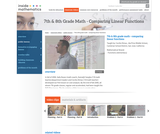Conditions of Use:
Read the Fine Print
Rating

The foundation of this lesson is constructing, communicating, and evaluating student-generated tables while making comparisons between three different financial plans. Students are given three different DVD rental plans and asked to analyze each one to see if they could determine when the 3 different DVD plans cost the same amount of money, if ever. (7th/8th Grade Math)

Subject:
Algebra
Material Type:
Activity/Lab
Lecture
Lesson Plan
Teaching/Learning Strategy
Provider:
Noyce Foundation
Provider Set:
Inside Mathematics
Author:
Dimas, Cecilio
Date Added:
11/30/2011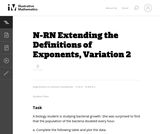Conditions of Use:
No Strings Attached
Rating

The goal of this task is to develop an understanding of why rational exponents are defined as they are (N-RN.1), however it also raises important issues about distinguishing between linear and exponential behavior (F-LE.1c) and it requires students to create an equation to model a context (A-CED.2

Subject:
Mathematics
Material Type:
Activity/Lab
Provider:
Illustrative Mathematics
Provider Set:
Illustrative Mathematics
Author:
Illustrative Mathematics
Date Added:
05/01/2012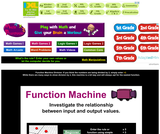Conditions of Use:
Read the Fine Print
Rating

Investigate the relationship between input and output values.

Subject:
Functions
Material Type:
Interactive
Provider:
Math Playground
Provider Set:
Individual Authors
Author:
Colleen King
Date Added:
08/11/2013
Remix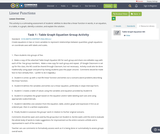Conditions of Use:
Remix and Share
Rating

This activity is a culmnating assessment of students' abilities to describe a linear function in words, in an equation, in a table, in a graph, identify a solution, and explain the solution.

Subject:
Educational Technology
Mathematics
Algebra
Material Type:
Lesson Plan
Author:
Shawn Dykstra
Date Added:
12/07/2017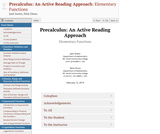Conditions of Use:
Remix and Share
Rating

This book is a collection of activities, a digital workbook, that replaces traditional textbook reading with an interactive, guided-lesson approach to learning. The workbook covers common topics of precalculus that are otherwise delivered as passive reading assignments or lectures.

Each chapter begins with activities which introduce students to the basics of a topic. Activities can be assigned, graded and recorded through the use of WeBWorK, an open-source online homework system. Many exercises include dynamic GeoGebra graphs for students to manipulate.

Following the activities, each chapter has a Gist section which resembles the expository content in a traditional textbook, though it still has interactive components such as found in the activities.

Subject:
Mathematics
Material Type:
Textbook
Provider:
Mt. Hood Community College
Author:
Jack Green
Nick Chura
Date Added:
03/03/2019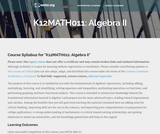Conditions of Use:
Read the Fine Print
Rating

This course is oriented toward US high school students. Mathematics comes together in this course. You enter precalculus with an abundant array of experience in mathematics, and this course offers an opportunity to make connections among the big ideas you encountered earlier. It also assists you in developing fluency with the tools used in learning calculus.

Subject:
Calculus
Functions
Material Type:
Full Course
Provider:
The Saylor Foundation
Date Added:
08/28/2013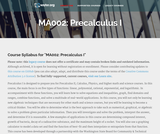Conditions of Use:
Read the Fine Print
Rating

Precalculus I is designed to prepare you for Precalculus II, Calculus, Physics, and higher math and science courses. In this course, the main focus is on five types of functions: linear, polynomial, rational, exponential, and logarithmic. In accompaniment with these functions, you will learn how to solve equations and inequalities, graph, find domains and ranges, combine functions, and solve a multitude of real-world applications.

Subject:
Calculus
Material Type:
Full Course
Provider:
The Saylor Foundation
Date Added:
08/28/2013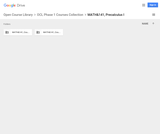Conditions of Use:
No Strings Attached
Rating

This course will cover families of functions, their properties, graphs and applications. These functions include: polynomial, rational, exponential, logarithmic functions and combinations of these. We will solve related equations and inequalities and conduct data analysis, introductory mathematical modeling and develop competency with a graphing calculator.Login: guest_oclPassword: ocl

Subject:
Functions
Material Type:
Full Course
Homework/Assignment
Lecture Notes
Lesson Plan
Reading
Syllabus
Provider:
Washington State Board for Community & Technical Colleges
Provider Set:
Open Course Library
Date Added:
10/31/2011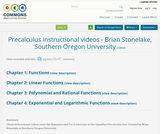Conditions of Use:
No Strings Attached
Rating

These instructional videos cover the Examples and Try It exercises in the OpenStax Precalculus text. Created by Brian Stonelake at Southern Oregon University.

Subject:
Mathematics
Material Type:
Lecture
Author:
Sonya Bennett-Brandt
Date Added:
09/06/2016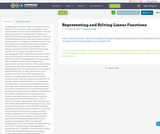Rating

In eighth grade, students studied how linear functions represented by equations, tables, and graphs can be used to model and solve real world situations. Particular attention was given to using the rate of change and initial value from multiple representations to model the relationship between two values with the generalizable function, y=mx+b. In Algebra 1, students continue using and making connections between representations of linear functions. A common misconception when making connections between representations is that constant rate of change and slope can be used interchangeably when “a linear function does not have slope, but the graph of a non-vertical line has a slope.” (High School Functions progression document, page 6) Unit 2 establishes a deep understanding of the characteristics of linear functions. This understanding includes exploring linear functions geometrically by analyzing the effects of transformations on the graph by replacing f(x) by f(x) + k, kf(x), f(kx) and f(x + k) for specific values of k. These understandings allow students to compare linearity to function families studied in future units (e.g., exponential, quadratic).

In this unit, students will extend their focus from slope intercept form to reasoning about standard form and point slope form. Students begin to make distinctions about which of these forms are most beneficial when modeling a real world situation. Different contexts lend themselves to different forms of linear equations. Students may build a function to model a situation, using parameters from that situation (e.g., rate of change, start value, ordered pair). Other situations are more efficiently modeled with standard form (e.g., Dana purchased 3 brauts and 4 drinks for \$8.50). Symbolic manipulation from one form to another can reveal new characteristics of the function or assist in solving systems of equations.

In 6th and 7th grade students solved one and two step equations and inequalities algebraically. In 8th grade students solved linear equations using graphs, tables, and algebraic manipulation. In this unit, students will apply what they know about solving equations and inequalities to solving multi-step inequalities which include variables on both sides. Students will make sense of what a solution means for an equation compared to an inequality. Students will extend their understanding of solving linear equations with two variables. First, they will manipulate equations to solve for specific variables. Second, students will justify their reasoning by supplying mathematical properties to explain each step in solving an equation. This work will help set the groundwork for mathematical proofs in tenth grade.

In addition, students will extend their 8th grade understanding of solving systems of linear equations to include systems of linear inequalities as representations of real world situations. Students will solve systems of linear equations exactly (e.g. with substitution principle, combination/elimination), and approximately (e.g., with graphs) with a new emphasis on the conceptual understanding and justification of why these strategies work. Students will compare and contrast the benefit of using each of these strategies in different situations.

In this unit students are using what they know about linear functions to build new understandings of piecewise linear functions including absolute value functions. In addition, students will use tables and graphs to solve absolute value equations as described in HSA.REI.D.11. The Michigan State Standards no longer require students to be able to algebraically solve piecewise and absolute value functions.

Subject:
Mathematics
Material Type:
Module
Unit of Study
Author:
Anupam Chugh
Date Added:
06/20/2017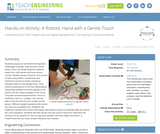Conditions of Use:
Read the Fine Print
Rating

Students groups act as NASA/GM engineers challenged to design, build and test robotic hands, which are tactile feedback systems made from cloth gloves and force sensor circuits. Student groups construct force sensor circuits using electric components and FlexiForce sensors to which resistance changes based on the applied force. They conduct experiments to find the mathematical relationship between the force applied to the sensor and the output voltages of the circuit. They take several measurements force vs. resistance, force vs. voltage and use the data to find the best fit curve models for the sensor. Different weights applied to the sensor are used as a scalable force. Students use traditional methods and current technology (calculators) to plot the collected data and define the curve equations. Students test their gloves and use a line of best fit to determine the minimum force required to crack an egg held between the index finger and thumb. A PowerPoint(TM) file and many student handouts are included.

Subject:
Engineering
Material Type:
Activity/Lab
Provider:
TeachEngineering
Provider Set:
TeachEngineering
Author:
Luis Avila
Date Added:
10/14/2015